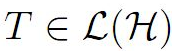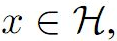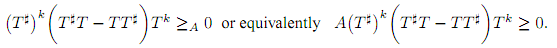The Australian Journal of Mathematical Analysis and Applications

 Home News Editors Volumes RGMIA Subscriptions Authors Contact

ISSN 1449-5910

Sorry death is imminent for file: ../public_html/searchroot/files/tex/v19n2/v19i2p4.tex
You searched for lohaj
Total of 12 results found in site

5: Paper Source PDF document

Paper's Title:

Some properties of k-quasi class Q* operators

Author(s):

Shqipe Lohaj and Valdete Rexhëbeqaj Hamiti

Department of Mathematics,
Faculty of Electrical and Computer Engineering,
University of Prishtina "Hasan Prishtina",
Prishtine 10000,
Kosova.
E-mail: shqipe.lohaj@uni-pr.edu

Department of Mathematics,
Faculty of Electrical and Computer Engineering,
University of Prishtina "Hasan Prishtina",
Prishtine 10000,
Kosova.
E-mail: valdete.rexhebeqaj@uni-pr.edu

Abstract:

In this paper, we give some results of k-quasi class Q* operators. We proved that if T is an invertible operator and N be an operator such that N commutes with T*T, then N is k-quasi class Q* if and only if TNT-1 is of k-quasi class Q*. With example we proved that exist an operator k-quasi class Q* which is quasi nilpotent but it is not quasi hyponormal.

3: Paper Source PDF document

Paper's Title:

Some properties of quasinormal, paranormal and 2-k* paranormal operators

Author(s):

Shqipe Lohaj

Department of Mathematics,
University of Prishtina,
10000, Kosova.
E-mail: shqipe.lohaj@uni-pr.edu

Abstract:

In the beginning of this paper some conditions under which an operator is partial isometry are given. Further, the class of 2-k* paranormal operators is defined and some properties of this class in Hilbert space are shown. It has been proved that an unitarily operator equivalent with an operator of a 2-k* paranormal operator is a 2-k* paranormal operator, and if is a 2-k* paranormal operator, that commutes with an isometric operator, then their product also is a \$2-k^*\$ paranormal operator.

3: Paper Source PDF document

Paper's Title:

Structural and Spectral Properties of k-Quasi Class Q Operators

Author(s):

Valdete Rexhëbeqaj Hamiti and Shqipe Lohaj

Department of Mathematics,
Faculty of Electrical and Computer Engineering,
University of Prishtina "Hasan Prishtina",
Prishtine 10000,
Kosova.
E-mail: valdete.rexhebeqaj@uni-pr.edu

Department of Mathematics,
Faculty of Electrical and Computer Engineering,
University of Prishtina "Hasan Prishtina",
Prishtine 10000,
Kosova.
E-mail: shqipe.lohaj@uni-pr.edu

Abstract:

An operatoris said to be k-quasi class Q if, for allwhere k is a natural number. In this paper, first we will prove some results for the matrix representation of k-quasi class Q operators. Then, we will give the inclusion of approximate point spectrum of k-quasi class Q operators. Also, we will give the equivalence between Aluthge transformation and *-Aluthge transformation of k-quasi class Q operators.

1: Paper Source PDF document

Paper's Title:

Hyponormal and K-Quasi-Hyponormal Operators On Semi-Hilbertian Spaces

Author(s):

Ould Ahmed Mahmoud Sid Ahmed and Abdelkader Benali

Mathematics Department,
College of Science,
Aljouf University,
Aljouf 2014,
Saudi Arabia.
E-mail: sididahmed@ju.edu.sa

Mathematics Department, Faculty of Science,
Hassiba Benbouali, University of Chlef,
B.P. 151 Hay Essalem, Chlef 02000,
Algeria.
E-mail: benali4848@gmail.com

Abstract:

Let H be a Hilbert space and let A be a positive bounded operator on H. The semi-inner product < u|v>A:=<Au|v>, u,v H induces a semi-norm || .||A on H. This makes H into a semi-Hilbertian space. In this paper we introduce the notions of hyponormalities and k-quasi-hyponormalities for operators on semi Hilbertian space (H,||.||A), based on the works that studied normal, isometry, unitary and partial isometries operators in these spaces. Also, we generalize some results which are already known for hyponormal and quasi-hyponormal operators. An operator T BA (H) is said to be (A, k)-quasi-hyponormal ifSearch and serve lasted 0 second(s).